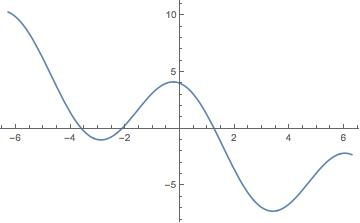# When do I use FindRoot instead of NSolve?

Like Solve, NSolve is designed primarily for solving polynomial equations.

``````In:= NSolve[4 - r^2 == r, r]
Out= {{r -> -2.56155}, {r -> 1.56155}}``````

The algebraic methods available in NSolve cannot handle general equations with transcendental functions—for example:

``````In:= NSolve[4 Cos[r] == r, r]
NSolve::nsmet: This system cannot be solved with the methods available to NSolve.
Out= NSolve[4 Cos[r] == r, r] ``````

FindRoot uses numerical methods for starting at an initial value for the independent variable and locating a solution:

``````In:= fr = FindRoot[ 4 Cos[r] == r, {r, 1}]
Out= {r -> 1.25235}  ``````

Verify that the returned value solves the equation:

``````In:= (4 Cos[r] - r) /. fr // Chop
Out= 0
``````

FindRoot finds one solution per evaluation. To obtain further solutions with FindRoot, initial values need to be chosen sufficiently close to the other root locations:

``````In:= Plot[ 4 Cos[r] - r, {r, -2 Pi, 2 Pi}]
````````````In:= FindRoot[ 4 Cos[r] == r, {r, -4}]
Out= {r -> -3.5953}``````

``````In:= FindRoot[ 4 Cos[r] == r, {r, -2}]
Out= {r -> -2.13333}
``````

## Contact SupportCall us

## Customer Support

Monday–Friday
8am–5pm US Central Time

• Product registration or activation
• Pre-sales information and ordering
• Help with installation and first launch

## Advanced Technical Support (for eligible customers)

Monday–Thursday
8am–5pm US Central Time

Friday
8:30–10am & 11am–5pm US Central Time

• Priority technical support
• Product assistance from Wolfram experts
• Help with Wolfram Language programming